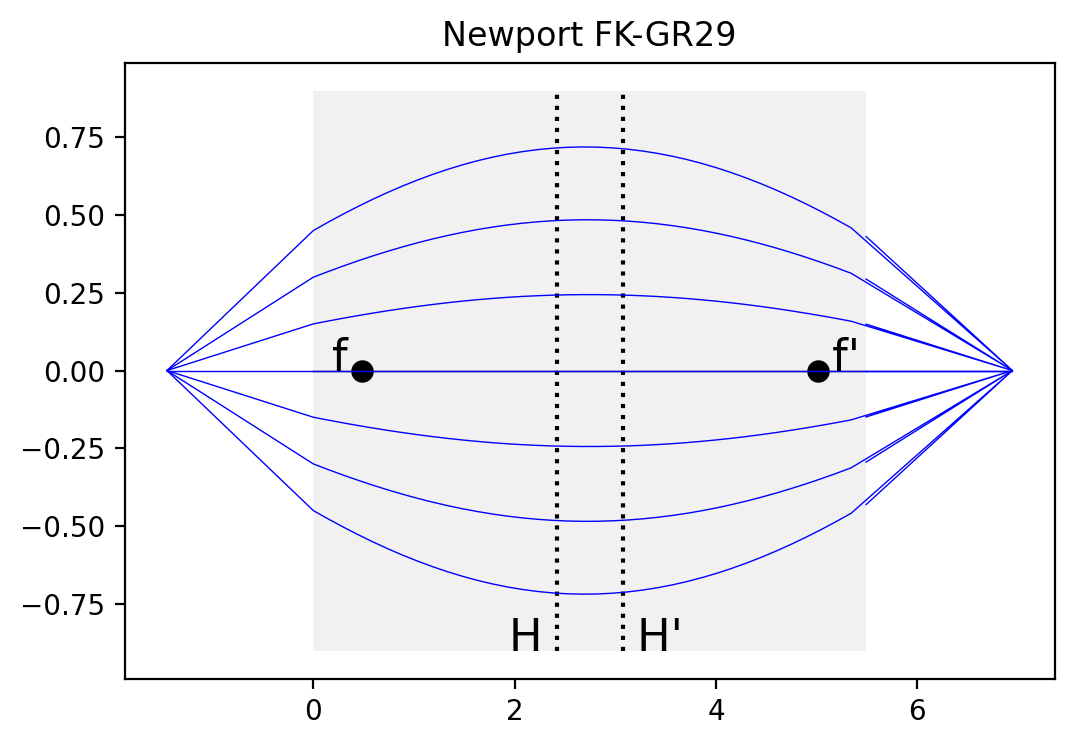# Gradient Index Lenses¶

Scott Prahl

May 2020

:

import sys
import numpy as np
import matplotlib.pyplot as plt
import matplotlib.patches as patches

try:
import pygrin
except:
print("***** You need to install the pygrin module first           *****")
print("***** Execute the following line in a new cell, then retry *****")
print()
print("!{sys.executable} -m pip install --user pygrin")


## Pitch of a GRIN lens¶

A gradient index (GRIN) lens is characterized by a sinusoidal ray path along the lens. A lens is typically characterized its pitch or the number of sinusoidal periods within the lens.

If the pitch is one (or an integer) then the grin lens acts as a relay and reproduces the light incident on the entrance surface at the exit surface. A half-pitch inverts the pattern. A quarter-pitch lens will collimate a point source or focus a collimated incident beam.

:

n0 = 1.608              # centerline index of refraction
length = 1              # mm
diameter = 0.25            # mm
angle = 40*np.pi/180 # radians
xpos = 0
pitch = 1

# max angle in air
max_angle = pygrin.max_angle(n0,pitch,length,diameter)
# range of launch angles
angles = np.linspace(-max_angle,max_angle,6)
# range of launch angles in grin lens
angles = np.arcsin(np.sin(angles/n0))

plt.subplots(2,1,figsize=(10,10))
plt.subplot(2,1,1)
for angle in angles:
z,r = pygrin.meridional_curve(n0, pitch, length, xpos, angle)
plt.plot(z,r, lw=0.5)

plt.xticks([0,0.25,0.5,1.0])
plt.yticks([])
plt.xlabel('Pitch')
plt.title('')

plt.subplot(2,1,2)
z,r = pygrin.meridional_curve(n0, pitch, length, xpos, 0)
plt.plot(z,r, lw=0.5)

plt.xticks([0,0.25,0.5,1.0])
plt.yticks([])
plt.xlabel('Pitch')
plt.title('')

plt.show()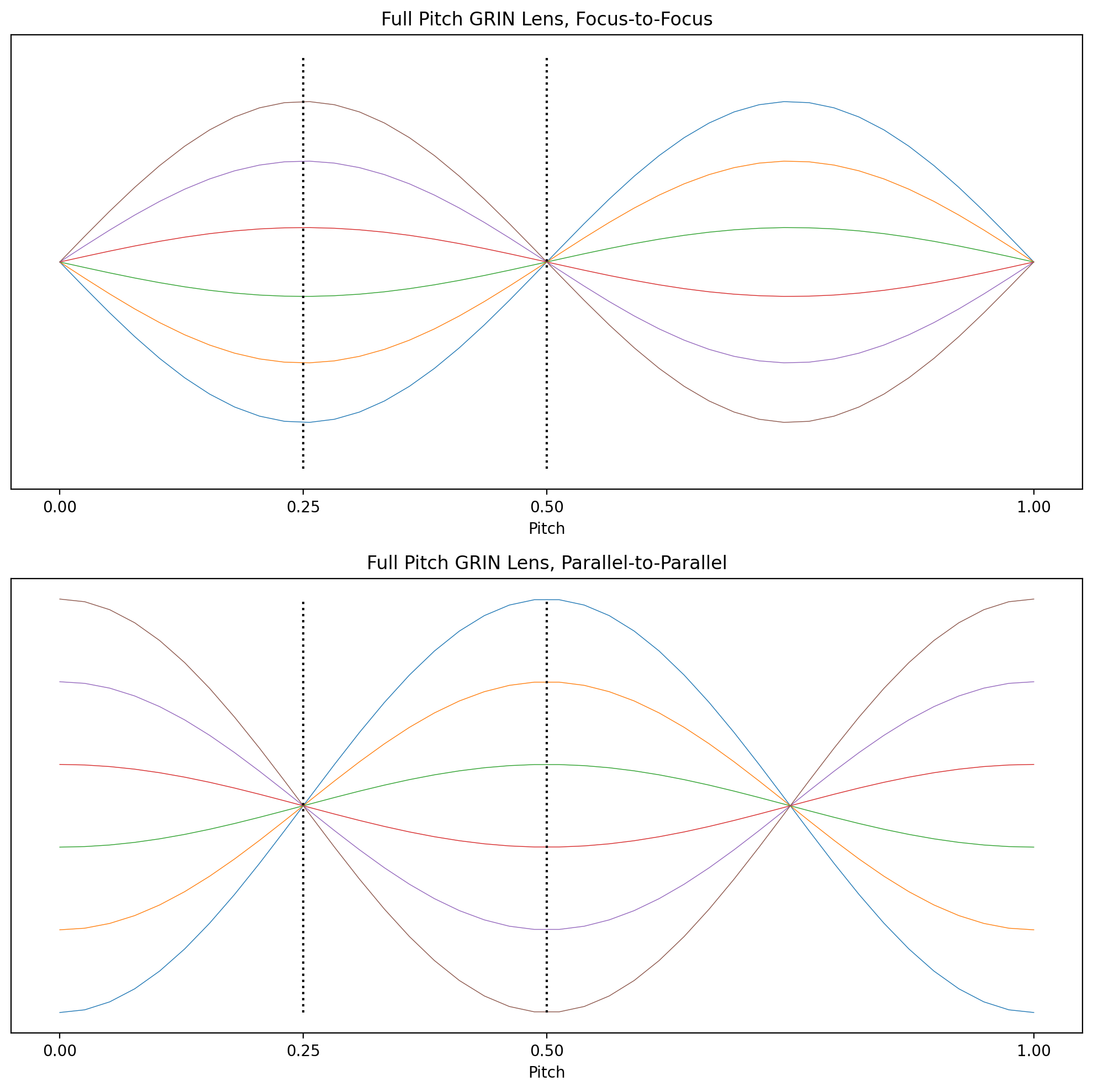### Quarter Pitch¶

This is the typical example because collimated light is focused to a point, or conversely, a point source is collimated. Here we see an example the former.

:

pitch = 0.25
n0 = 1.608              # centerline index of refraction
length = 5              # mm
diameter = 2            # mm
angle = 0*np.pi/180     # radians

pygrin.plot_principal_planes(n0, pitch, length, diameter)
xpos = np.linspace(-diameter/2,diameter/2,9)
for pos in xpos:
z,r = pygrin.meridional_curve(n0, pitch, length, pos, angle)
plt.plot(z,r, color='blue',lw=0.5)

plt.rcParams["figure.figsize"] = [10,3]
plt.axis('off')
plt.show()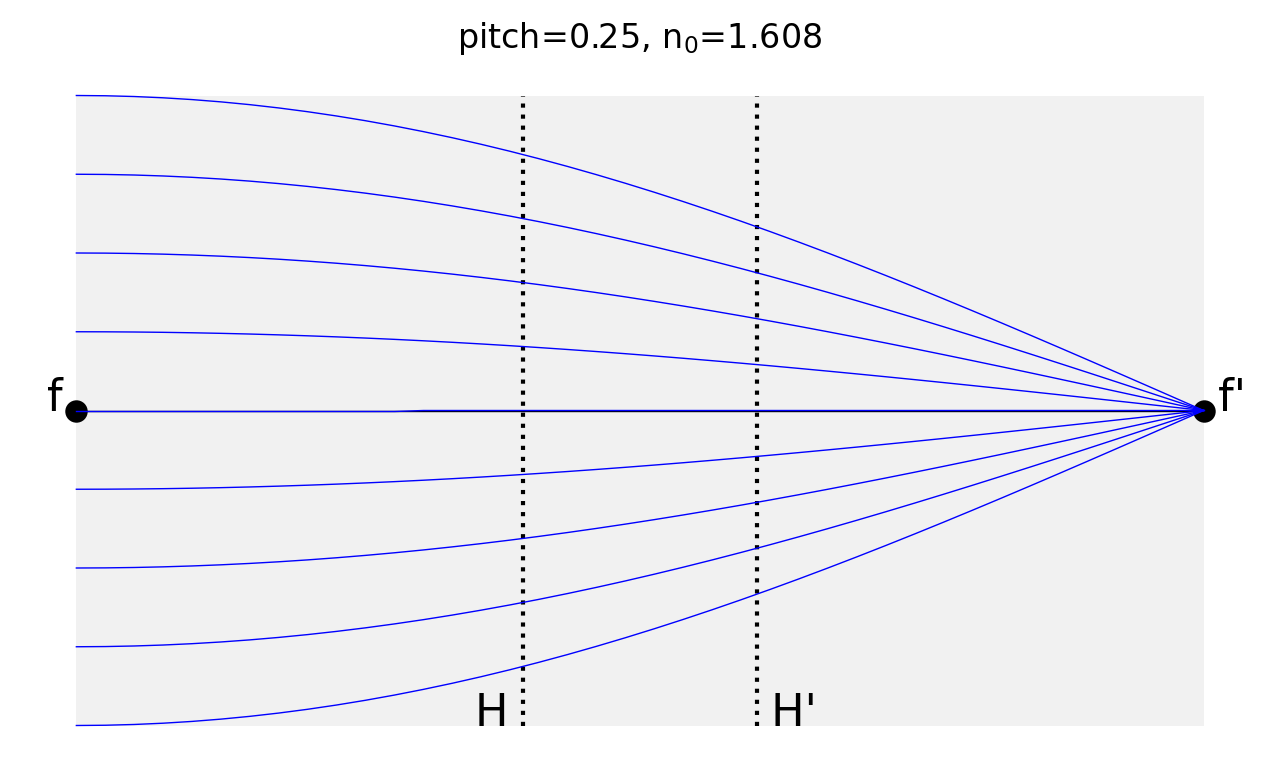This shows light being collimated from a point source

:

pitch = 0.25
n0 = 1.608              # centerline index of refraction
length = 5              # mm
diameter = 2            # mm
xpos = 0                # mm

pygrin.plot_principal_planes(n0, pitch, length, diameter)

# max angle in air
max_angle = pygrin.max_angle(n0,pitch,length,diameter)
# range of launch angles
angles = np.linspace(-max_angle,max_angle,9)
# range of launch angles in grin lens
angles = np.arcsin(np.sin(angles/n0))

for angle in angles:
z,r = pygrin.meridional_curve(n0, pitch, length, xpos, angle)
plt.plot(z,r, color='blue',lw=0.5)

plt.rcParams["figure.figsize"] = [10,3]
plt.axis('off')
plt.show()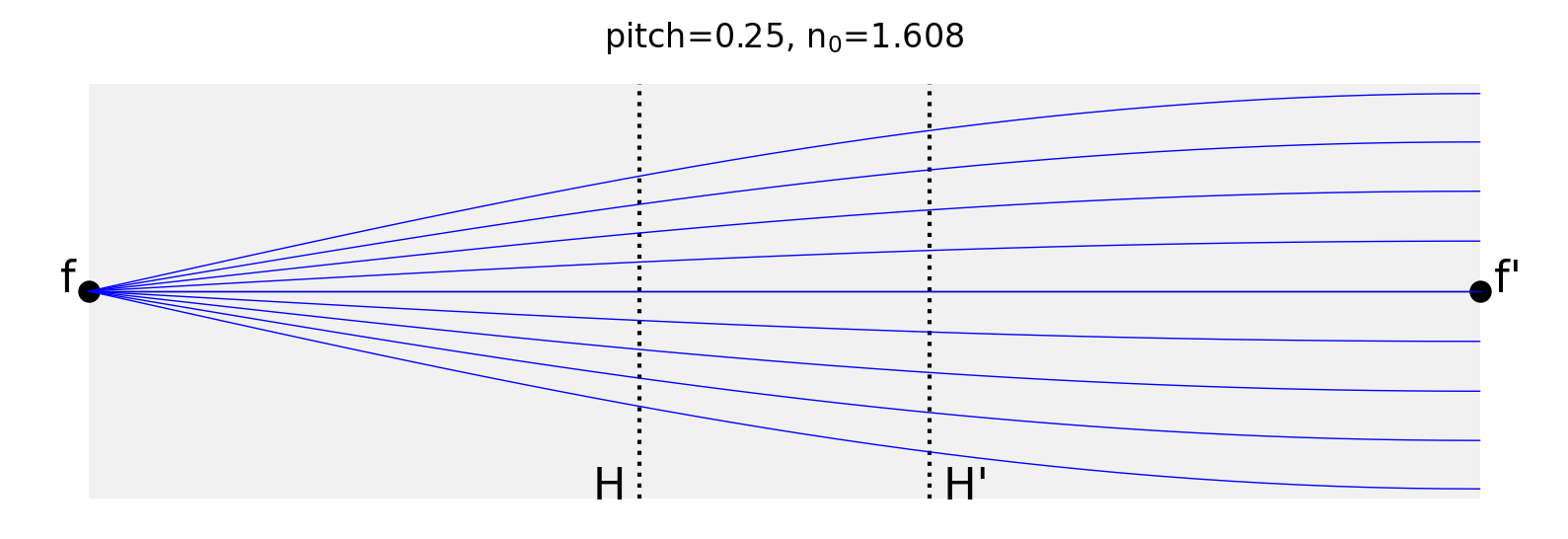Here is a 4f system. Here the source on the left is on focal distance from the front face and the

:

# 4f system
pitch = 0.25
n0 = 1.608              # centerline index of refraction
length = 5              # mm
diameter = 2            # mm
zobj = pygrin.EFL(n0,pitch,length)
xpos = np.linspace(-diameter/4,diameter/4,9)

pygrin.plot_principal_planes(n0, pitch, length, diameter)

for pos in xpos:
z,r = pygrin.full_meridional_curve(n0, pitch, length, -zobj, 0.0, pos)
plt.plot(z,r, color='blue',lw=0.5)

plt.rcParams["figure.figsize"] = [10,3]
plt.axis('off')
plt.show()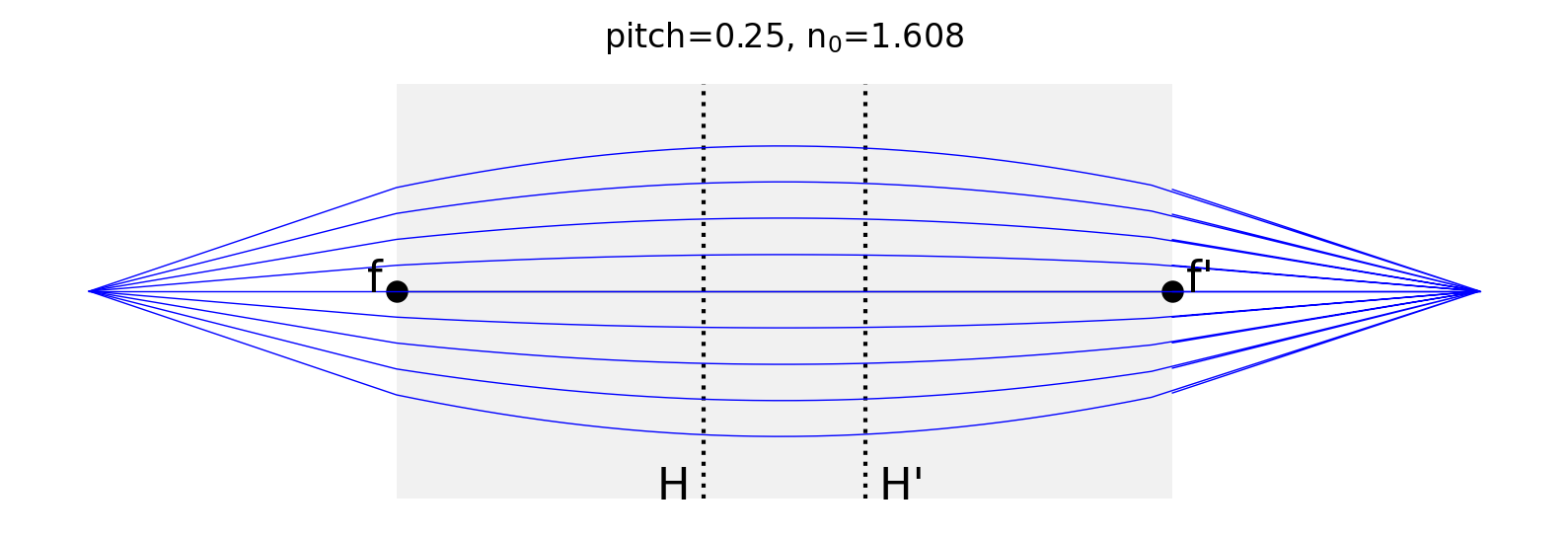Finally, this shows that collimated light incident at an angle on the lens will be imaged to a point off-axis.

:

# off axis launch
pitch = 0.25
n0 = 1.608              # centerline index of refraction
length = 5           # mm
diameter = 2          # mm
zobj = pygrin.EFL(n0,pitch,length)
xpos = np.linspace(-diameter/4,diameter/4,9)

pygrin.plot_principal_planes(n0, pitch, length, diameter)

for pos in xpos:
z,r = pygrin.full_meridional_curve(n0, pitch, length, -zobj, pos-0.1, pos)
plt.plot(z,r, color='blue',lw=0.5)

plt.rcParams["figure.figsize"] = [10,3]
plt.axis('off')
plt.show()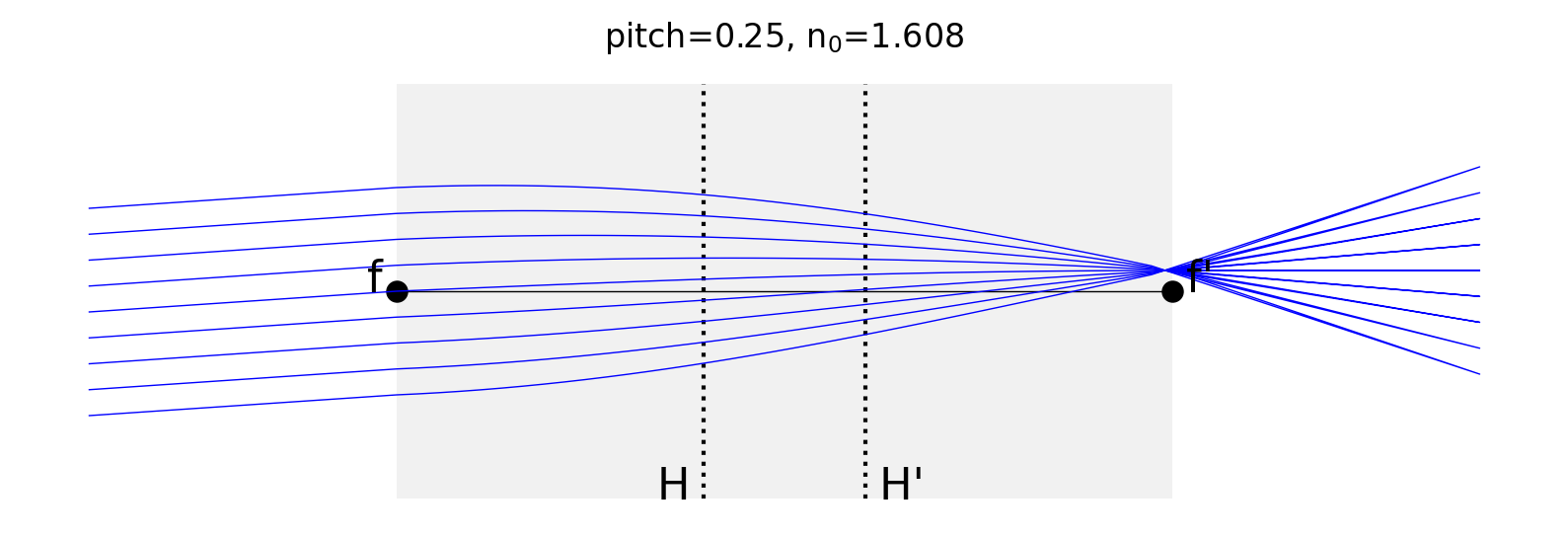### Half Pitch¶

Here we show that all the rays hitting the front surface at a point are imaged to the same point on the exit surface — but inverted.

The prinicipal planes are not drawn because the effective focal length is infinite.

:

pitch = 0.5
n0 = 1.608              # centerline index of refraction
length = 5              # mm
diameter = 2            # mm
xpos = 0.5                # mm

pygrin.plot_principal_planes(n0, pitch, length, diameter)

# max angle in air
max_angle = pygrin.max_angle(n0,pitch,length,diameter)
# range of launch angles
angles = np.linspace(-max_angle,max_angle,9)
# range of launch angles in grin lens
angles = np.arcsin(np.sin(angles/n0))

for angle in angles:
z,r = pygrin.meridional_curve(n0, pitch, length, xpos, angle)
plt.plot(z,r, color='blue',lw=0.5)

plt.rcParams["figure.figsize"] = [10,3]
plt.axis('off')
plt.show()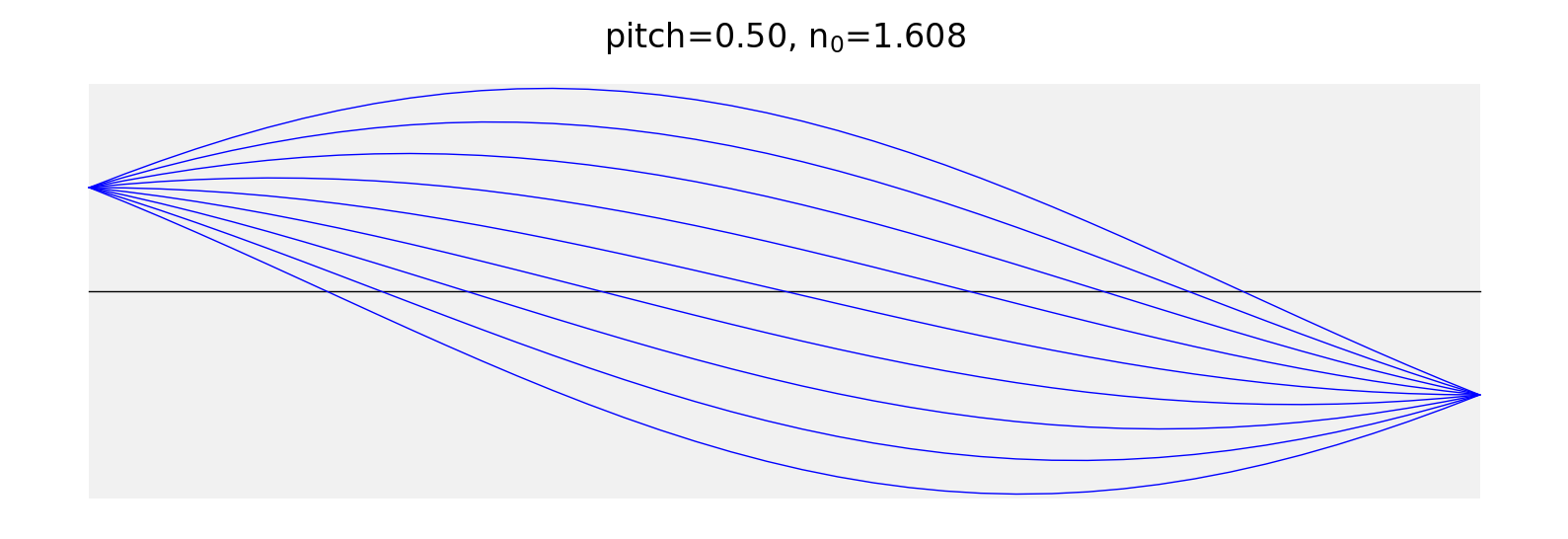# Index of Refraction Across Lens¶

## Parabolic Profile¶

:

n0 = 1.608
pitch = 0.25
length = 5    # mm
diameter = 2
r = np.linspace(-diameter/2,diameter/2,50)

plt.plot(r,pygrin.parabolic_profile_index(n0,pitch,length,r))
plt.plot([0,0],[1.53,n0],':k')
plt.ylabel('Index of Refraction')
plt.title(r'pitch=%.2f, n$_0$=%.3f, length=%.2fmm'%(pitch,n0,length))
plt.rcParams["figure.figsize"] = [6,4]

plt.show()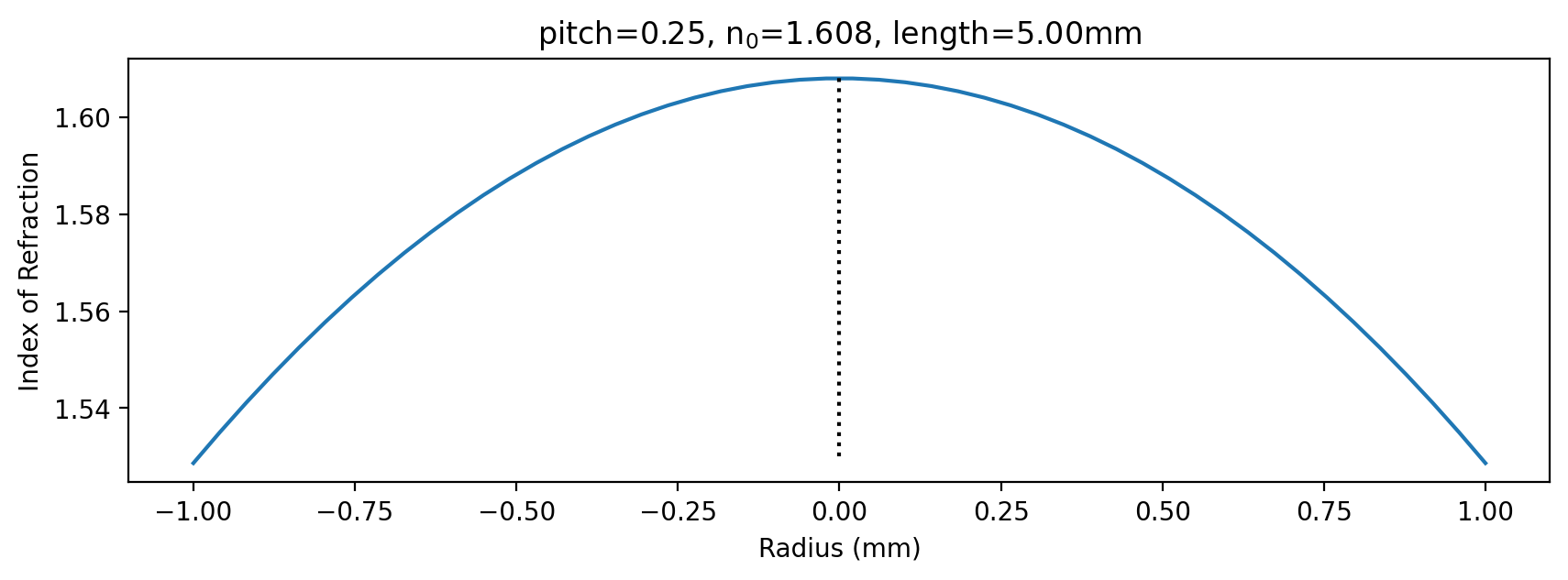## Hyperbolic Secant Profile¶

This has a few advantages over the parabolic profile: the propagation for a HS grin lens can be solved exactly and there aren’t any aberrations for meridional rays.

As you can see below, the HS profile can be quite close to the parabolic profile.

:

n0 = 1.608
pitch = 0.19
length = 5    # mm
diameter = 2

r = np.linspace(-diameter/2,diameter/2,50)

plt.subplots(2,1, sharex=True,figsize=(6,6))
plt.subplot(2,1,1)
n_p = pygrin.parabolic_profile_index(n0,pitch,length,r)
n_s = pygrin.hyperbolic_secant_profile_index(n0,alpha,r)
plt.plot(r,n_p)
plt.plot(r,n_s)
plt.xticks([])
plt.ylabel('Index of Refraction')
plt.title(r'pitch=%.2f, n$_0$=%.3f, length=%.2fmm'%(pitch,n0,length))
plt.rcParams["figure.figsize"] = [6,4]

plt.subplot(2,1,2)
plt.plot(r,n_p-n_s)

plt.ylabel('Difference')
plt.plot([-1,1],[0,0],':k')

plt.show()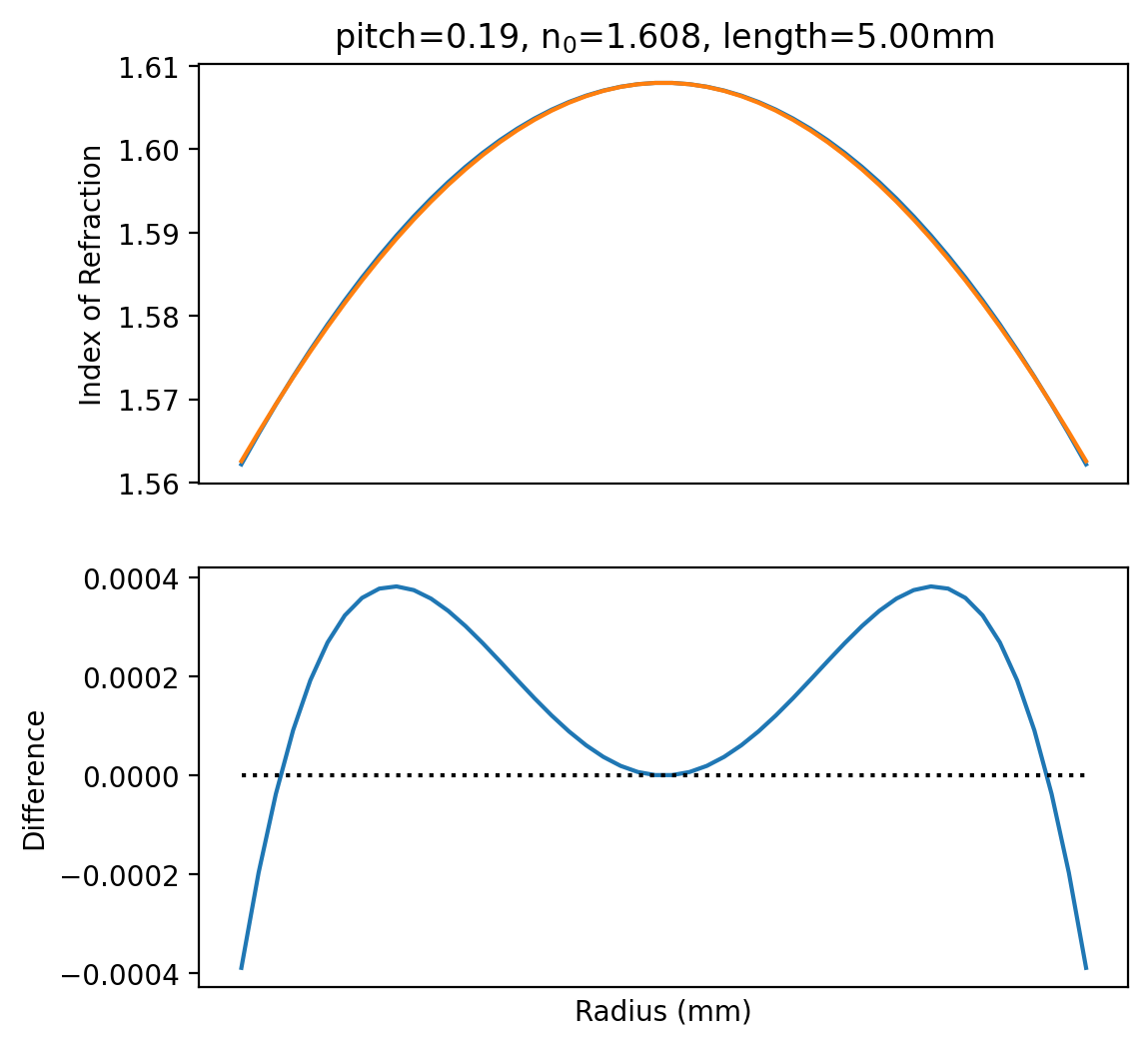# Catalog Examples¶

## Grin Lens from ancient Melles Griot Catalog, 4.67 line 2¶

Part number LGS-0.25-1.0-2.58-633

:

n = 1.608
length = 2.58
diameter = 1

pitch = pygrin.period(gradient, length)
print('expected pitch = 0.25,           calculated %.2f'%pitch)

efl = pygrin.EFL(n,pitch,length)
na = pygrin.NA(n,pitch,length,diameter)
print('expected NA = 0.46,              calculated %.2f'%na)

angle = pygrin.max_angle(n,pitch,length,diameter)
print('expected full accept angle = 55°, calculated %.0f°'%(2*angle*180/np.pi))

expected pitch = 0.25,           calculated 0.25
expected NA = 0.46,              calculated 0.46
expected full accept angle = 55°, calculated 54°

:

r = np.linspace(-0.5,0.5,50)
plt.plot(r,pygrin.parabolic_profile_index(n,pitch,length,r))
plt.ylabel('Index of Refraction')
plt.title('pitch=%.3f, n0=%.3f, L=%.2fmm'%(pitch,n,length))
plt.show()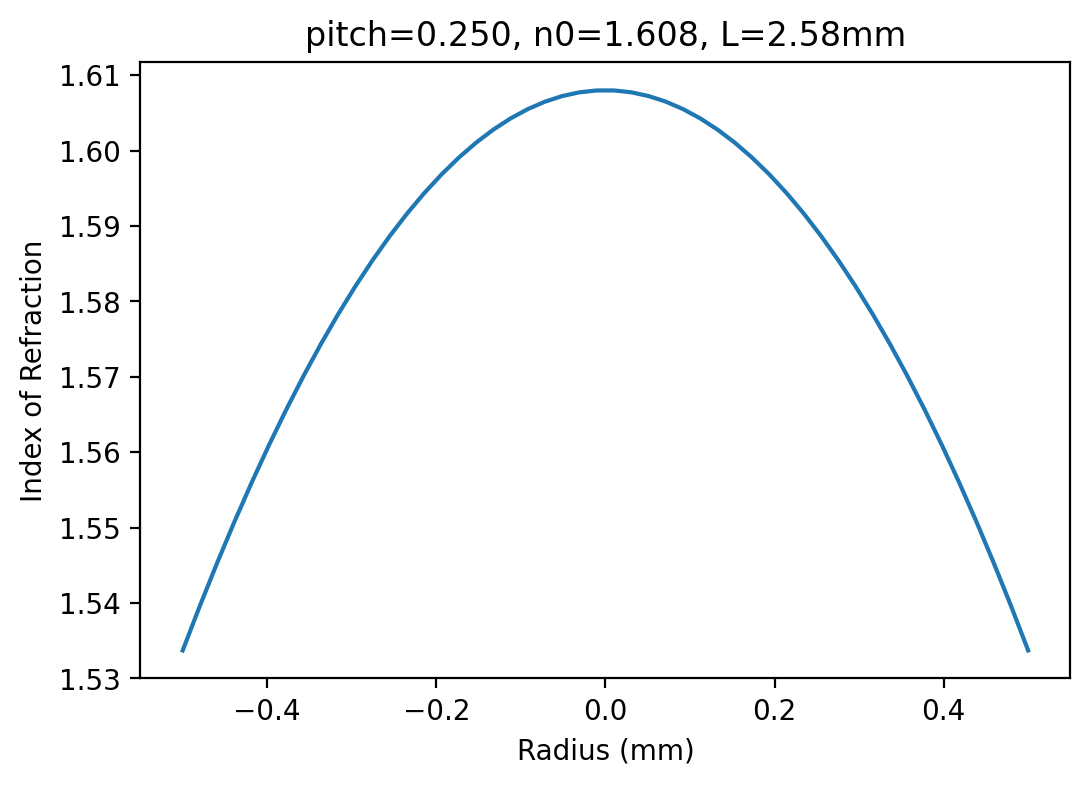:

pygrin.plot_principal_planes(n, pitch, length, diameter)
xpos = np.linspace(-diameter/2,diameter/2,7)
for pos in xpos:
z,r = pygrin.meridional_curve(n, pitch, length, pos, 0*np.pi/180)
plt.plot(z,r, color='blue',lw=0.5)

plt.title('Melles-Griot LGS-0.25-1.0-2.58-633')
plt.show()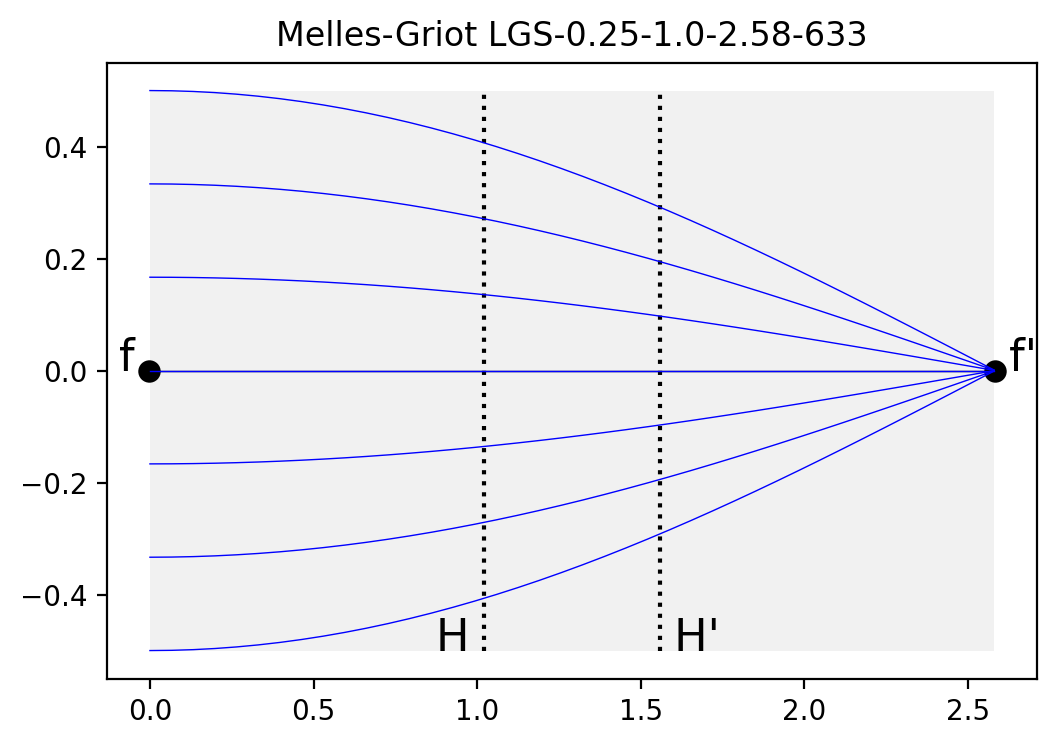## Grin Lens from ancient Melles Griot Catalog, 4.67 line 5¶

Part number LGE-0.29-1.8-5.37-633

:

n = 1.608
length = 5.37
diameter = 1.8

pitch = pygrin.period(gradient, length)
print('expected pitch = 0.29,            calculated %.2f'%pitch)

ffl = pygrin.FFL(n,pitch,length)
efl = pygrin.EFL(n,pitch,length)
print('expected FFL = 0.46,              calculated %.2f'%ffl)

na = pygrin.NA(n,pitch,length,diameter)
print('expected NA = 0.46,               calculated %.2f'%na)

angle = pygrin.max_angle(n,pitch,length,diameter)
print('expected full accept angle = 55°, calculated %.0f°'%(2*angle*180/np.pi))

print('working distance = %.2f mm'%(efl-ffl))

expected pitch = 0.29,            calculated 0.29
expected FFL = 0.46,              calculated 0.47
expected NA = 0.46,               calculated 0.46
expected full accept angle = 55°, calculated 55°
working distance = 1.43 mm

:

pygrin.plot_principal_planes(n, pitch, length, diameter)
xpos = np.linspace(-diameter/4, diameter/4,8)
for pos in xpos:
z,r = pygrin.full_meridional_curve(n, pitch, length, ffl-efl, 0, pos)
plt.plot(z,r, color='blue', lw=0.5)

plt.title('Melles-Griot LGE-0.29-1.8-5.37-633')

plt.show()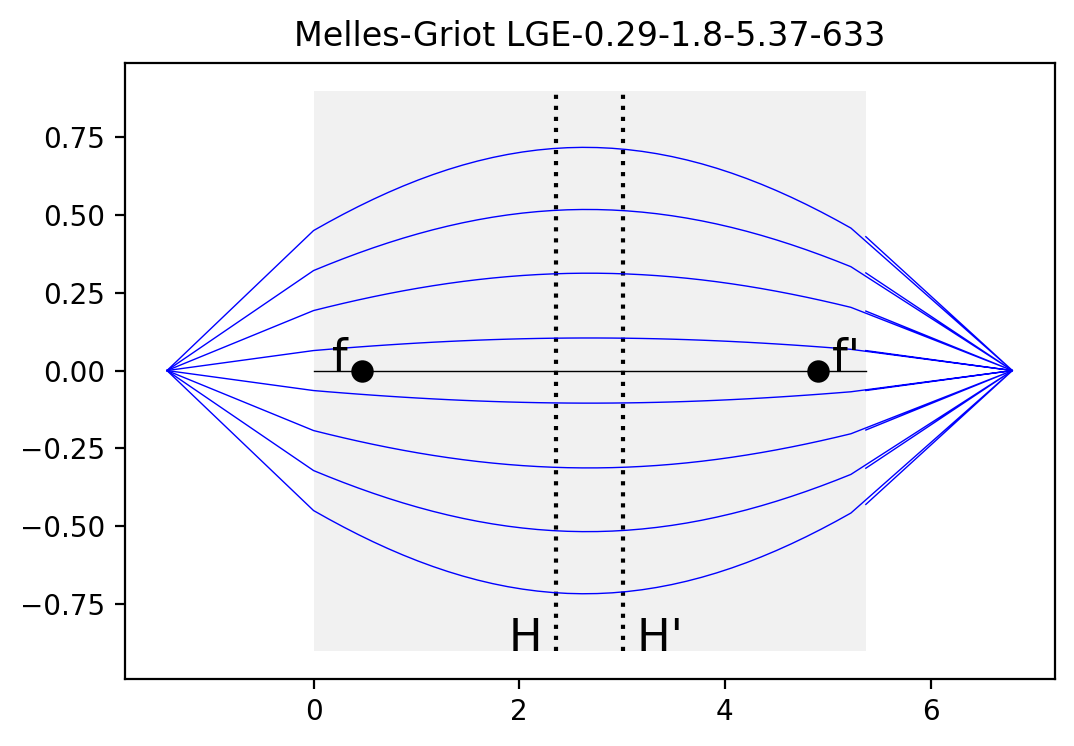## Riedl, page 96¶

:

n = 1.5834
length = 4
diameter = 1.8

pitch = pygrin.period(gradient, length)
print('expected pitch = 0.207,          calculated %.3f'%pitch)

efl = pygrin.EFL(n,pitch,length)
print('expected EFL = 2.00,             calculated %.2f'%efl)

ffl = pygrin.FFL(n,pitch,length)
print('expected FFL = -0.52,            calculated %.2f'%ffl)

bfl = pygrin.BFL(n,pitch,length)
print('expected BFL = 4.52,             calculated %.2f'%bfl)

na = pygrin.NA(n,pitch,length,diameter)
print('expected NA = 0.46,              calculated %.2f'%na)

angle = pygrin.max_angle(n,pitch,length,diameter)
print('expected full accept angle = 55°, calculated %.0f°'%(2*angle*180/np.pi))

print('working distance = %.2f mm'%(-ffl+efl))

expected pitch = 0.207,          calculated 0.208
expected EFL = 2.00,             calculated 2.00
expected FFL = -0.52,            calculated -0.52
expected BFL = 4.52,             calculated 4.52
expected NA = 0.46,              calculated 0.44
expected full accept angle = 55°, calculated 52°
working distance = 2.53 mm

:

pygrin.plot_principal_planes(n, pitch, length, diameter)
xpos = np.linspace(-diameter/2, diameter/2,7)
for pos in xpos:
z,r = pygrin.full_meridional_curve(n, pitch, length, ffl-efl, pos, pos)
plt.plot(z,r, color='blue', lw=0.5)

plt.show()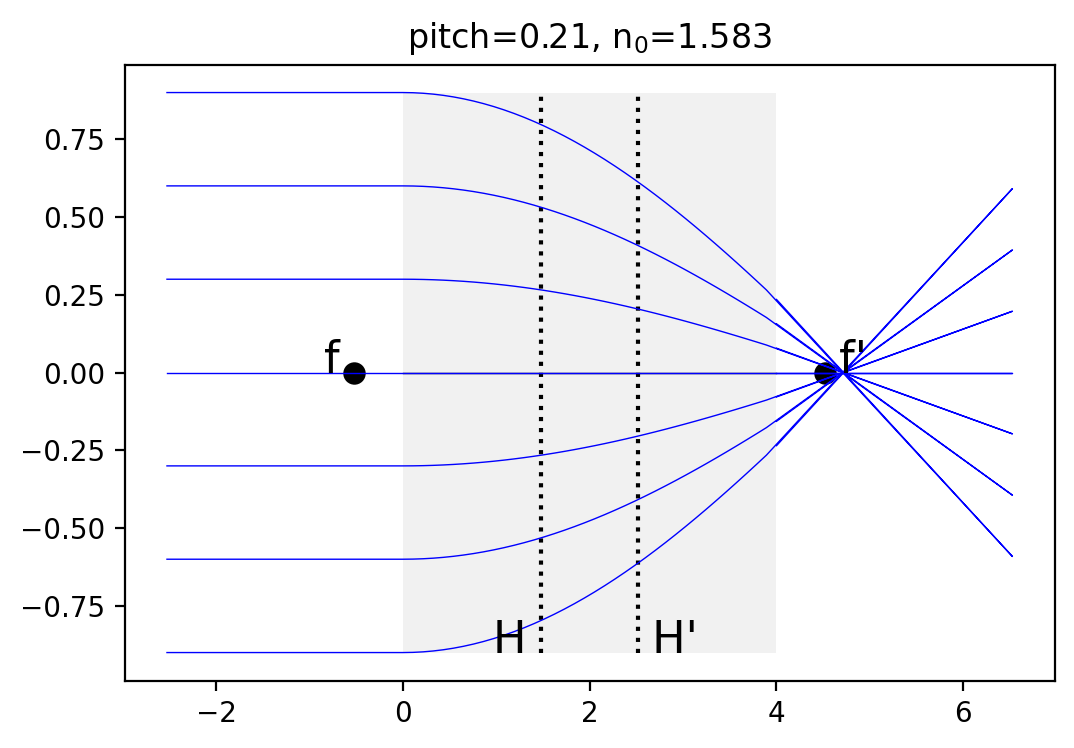# Grin Lenses at Oregon Tech¶

## Oriel 41425¶

Designed for 632.8nm

:

n = 1.608
pitch = 0.25
length = 6.28
diameter = 2

print('expected gradient = 0.250,        calculated %.3f'%pitch)

efl = pygrin.EFL(n,pitch,length)
#print('expected EFL = 2.00,              calculated %.2f'%efl)

ffl = pygrin.FFL(n,pitch,length)
#print('expected FFL = -0.52,             calculated %.2f'%ffl)

bfl = pygrin.BFL(n,pitch,length)
#print('expected BFL = 4.52,              calculated %.2f'%bfl)

na = pygrin.NA(n,pitch,length,diameter)
print('expected NA = 0.38,               calculated %.2f'%na)

angle = pygrin.max_angle(n,pitch,length,diameter)
print('expected full accept angle = 45°, calculated %.0f°'%(2*angle*180/np.pi))

print('working distance = %.2f mm'%(efl-ffl))

expected gradient = 0.250,        calculated 0.250
expected NA = 0.38,               calculated 0.38
expected full accept angle = 45°, calculated 45°
working distance = 2.49 mm

:

pygrin.plot_principal_planes(n, pitch, length, diameter)
xpos = np.linspace(-diameter/2, diameter/2,7)
for pos in xpos:
z,r = pygrin.full_meridional_curve(n, pitch, length, ffl-efl, pos, pos)
plt.plot(z,r, color='blue', lw=0.5)

plt.title('Oriel 41425')

plt.show()## Oriel 41440¶

Designed for 632.8nm

:

n = 1.608
pitch = 0.29
length = 5.35
diameter = 2

print('expected gradient = 0.290,        calculated %.3f'%pitch)

efl = pygrin.EFL(n,pitch,length)
#print('expected EFL = 2.00,              calculated %.2f'%efl)

ffl = pygrin.FFL(n,pitch,length)
#print('expected FFL = -0.52,             calculated %.2f'%ffl)

bfl = pygrin.BFL(n,pitch,length)
#print('expected BFL = 4.52,              calculated %.2f'%bfl)

na = pygrin.NA(n,pitch,length,diameter)
print('expected NA = 0.38,               calculated %.2f'%na)

angle = pygrin.max_angle(n,pitch,length,diameter)
print('expected full accept angle = 60°, calculated %.0f°'%(2*angle*180/np.pi))

print('working distance = %.2f mm'%(efl-ffl))

expected gradient = 0.290,        calculated 0.290
expected NA = 0.38,               calculated 0.50
expected full accept angle = 60°, calculated 60°
working distance = 1.42 mm

:

pygrin.plot_principal_planes(n, pitch, length, diameter)
xpos = np.linspace(-diameter/4, diameter/4,7)
for pos in xpos:
z,r = pygrin.full_meridional_curve(n, pitch, length, ffl-efl, 0, pos)
plt.plot(z,r, color='blue', lw=0.5)

plt.title('Oriel 41440')
plt.show()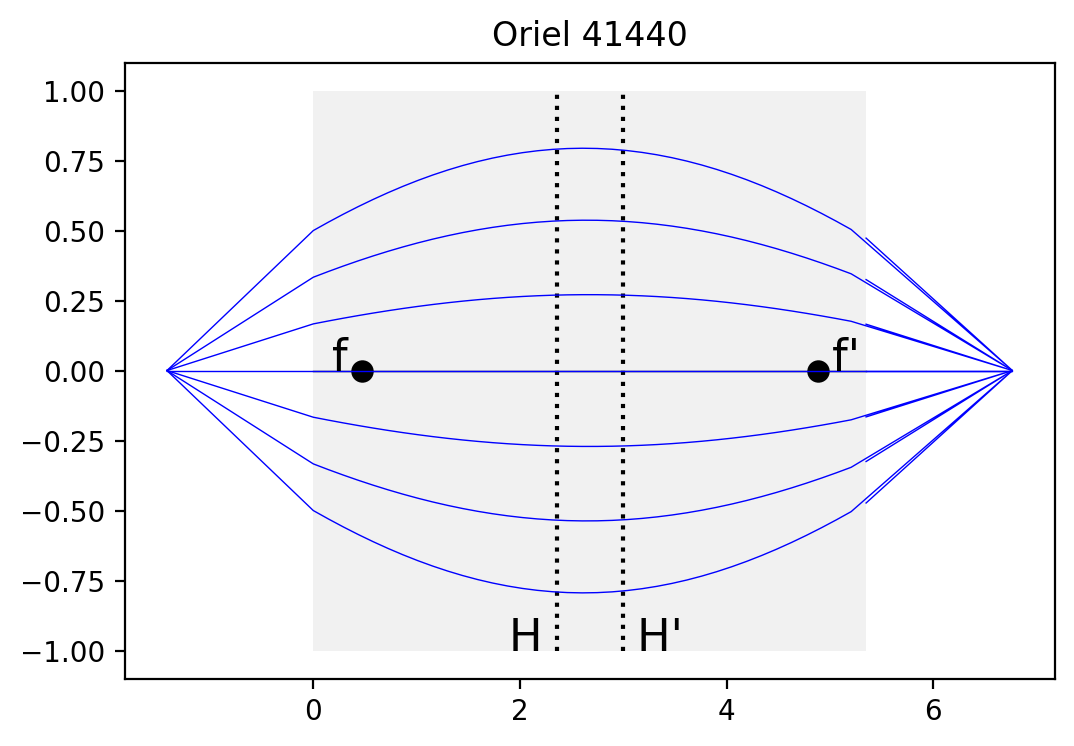## Newport FK - GR29¶

Designed for 850nm

:

n = 1.608
pitch = 0.29
length = 5.49
diameter = 1.8

print('expected gradient = 0.290,        calculated %.3f'%pitch)

efl = pygrin.EFL(n,pitch,length)
print('expected EFL = 1.95,              calculated %.2f'%efl)

ffl = pygrin.FFL(n,pitch,length)
#print('expected FFL = -0.52,             calculated %.2f'%ffl)

bfl = pygrin.BFL(n,pitch,length)
#print('expected BFL = 4.52,              calculated %.2f'%bfl)

na = pygrin.NA(n,pitch,length,diameter)
print('expected NA = 0.46,               calculated %.2f'%na)

angle = pygrin.max_angle(n,pitch,length,diameter)
print('expected full accept angle = 60°, calculated %.0f°'%(2*angle*180/np.pi))

print('working distance = %.2f mm'%(efl-ffl))

expected gradient = 0.290,        calculated 0.290
expected EFL = 1.95,              calculated 1.93
expected NA = 0.46,               calculated 0.45
expected full accept angle = 60°, calculated 53°
working distance = 1.45 mm

:

pygrin.plot_principal_planes(n, pitch, length, diameter)
xpos = np.linspace(-diameter/4, diameter/4,7)
for pos in xpos:
z,r = pygrin.full_meridional_curve(n, pitch, length, ffl-efl, 0, pos)
plt.plot(z,r, color='blue', lw=0.5)

plt.title('Newport FK-GR29')
plt.show()